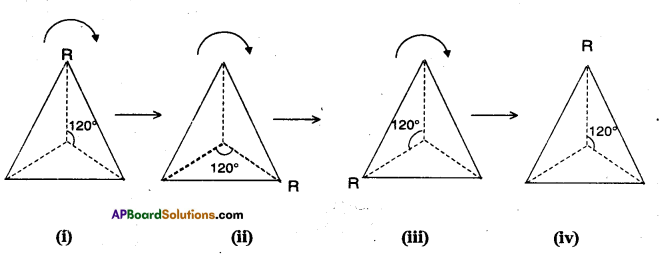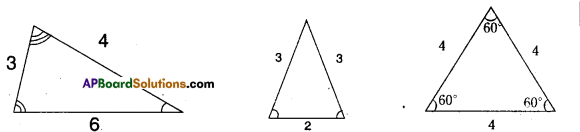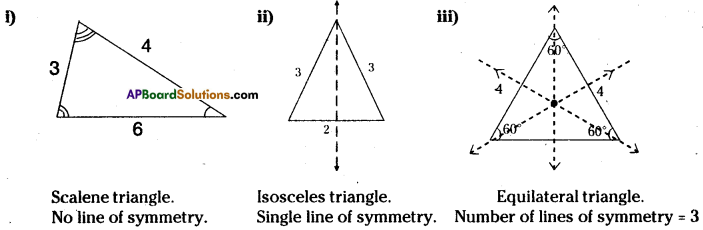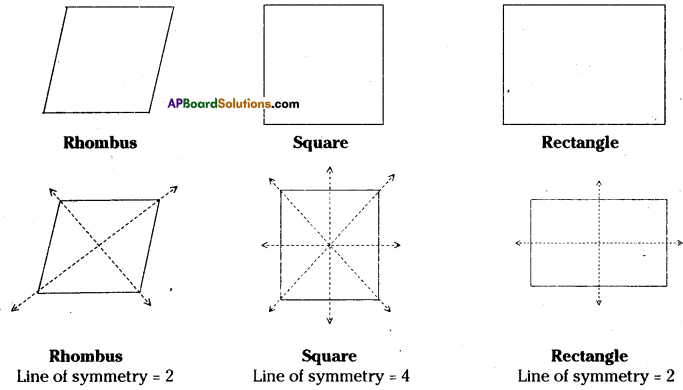AP State Syllabus AP Board 7th Class Maths Solutions Chapter 15 Symmetry InText Questions and Answers.

## AP State Syllabus 7th Class Maths Solutions 15th Lesson Chapter 15 Symmetry InText QuestionsDo This

Question 1.
What is the angle of rotational symmetry of a square ? (Page No. 285)
Solution:
90°

Question 2.
What is the angle of rotational symmetry of a parallelogram ? (Page No. 285)
Solution:
180°Question 3.
What is the angle of rotational symmetry of a circle ? (Page No. 285)
Solution:
The circle can be rotated through any angle to get rotational symmetry.

Try This

Question 1.
Name a few things in nature, that are symmetric. (Page No. 278)
Solution:
The things which have symmetry in nature are

1. An apple.
2. The Moon, the Sun and the Earth.
3. Head (face) of a tiger.
4. A human being face.
5. A rose flower.

Question 2.
Name 5 man made things that are symmetric. (Page No. 278)
Solution:

1. Awheel.
2. Square shaped cake.
3. A tube.
4. A Maths textbook.
5. A Cricket ball.Question 3.
i) Can you now tell the order of rotational symmetry for an equilateral triangle. (Page No. 285)ii) How many lines of symmetry ?
iii) What is the angle between every adjacent axes ?
Solution:
i) Order of rotational symmetry for an equilateral triangle = 120°
ii) 3 lines.
iii)120°

Question 4.
Look around you. Name five objects which have rotational symmetry (i.e rational symmetry of order more than 1). (Page No. 285)
Solution:
Circle, wheel, square etc.

Try This

Question 1.
Can we make a polygon with less than three line segments ? (Page No. 279)
Solution:
No. We can t make a polygon with less than three line segments.

Question 2.
What is the minimum number of sides of a polygon ? (Page No. 279)
Solution:
Minimum number of sides of a polygon is 3.

Question 3.
Given below are three types of triangles. Do all the triangles have the same number of lines of symmetry ? Which triangles has more ? (Page No. 279)Solution:An equilateral triangle has more number of lines of symmetry.Question 4.
Given below are different types of quadrilaterals. Do all of them have the same number of lines of symmetry ? Which quadrilateral has the most ? (Page No. 281)
Solution:By folding also we conclude that a regular polygon has the maximum number of lines / axes of symmetry.
In the above case a square has maximum number of axes of symmetry.
All of them do not have the same number of axes of symmetry.